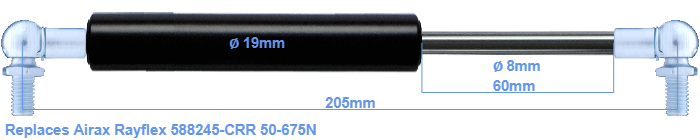# Replacement for Airax Rayflex 588245-CRR 50-675N

\$33.14

Replacement gas spring for the Airax Rayflex 588245-CRR 50-675 Newton. This fits a (possibly already present) ball with a diameter of 10mm. A corresponding ball is included. Brand: HAHN, a Stabilus company.

Please note:
The diameter of the tube of this replacement gas spring is 19mm instead of 20mm.
 Force Choose an option50 Newton60 Newton80 Newton100 Newton120 Newton140 Newton150 Newton160 Newton180 Newton200 Newton220 Newton240 Newton250 Newton260 Newton280 Newton300 Newton320 Newton340 Newton350 Newton360 Newton380 Newton400 Newton420 Newton440 Newton450 Newton460 Newton480 Newton500 Newton520 Newton540 Newton550 Newton560 Newton580 Newton600 Newton620 Newton640 Newton650 Newton660 Newton675 NewtonClear
This gas spring is also known as 5000588245-CRR, 588245-CRR.00.The diameter of the black cylinder of this gas spring is 19 millimeter. The cross section of the rod is 8 mm. The stroke of the gas spring is 60 millimeter. The total length of this replacement is 205 millimeter. This is the total distance between the centers of the two mounting parts. Without the mounting parts this gas spring is 165 mm long (thread to thread). The force of this replacement is 50-675 Newton. This is not an official Airax Rayflex gas spring, but a so called replacement. This gas spring was produced by Stabilus subsidiary HAHN. Nevertheless, the dimensions and force are almost entirely equal.
Category: